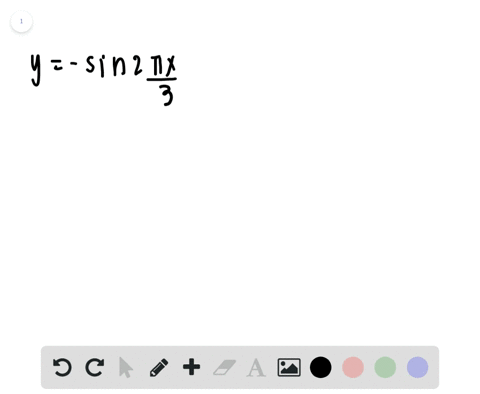Black Friday is Here! Start Your Numerade Subscription for 50% Off!Join Today### Sketching the Graph of a Trigonometric Function I…

00:39University of Southern California
Problem 56

# Sketching the Graph of a Trigonometric Function In Exercises $55-66,$ sketch the graph of the function.$$y=2 \cos 2 x$$

## Discussion

You must be signed in to discuss.

## Video Transcript

the number 56 asks us to make a sketch of the following triggered a metric function. Why equals to co sign of two X. So, in order to graft this function, we need to first gain two critical pieces of information. We need to know the amplitude of our function and the period. And we can obtain those two pieces easily just by looking at, ah, our equation of here because we know that for any co sign function in this form, the amplitude is always equal to a and in this case, a equals two, which means that our function is gonna have an amplitude of two. Now for the period, we're gonna look to that be term, and we know that the period is the same as two pi divided by the absolute value would be so appear we see that b equals two, and by dividing two pi divided by two our periods pie. So essentially are co sign function is gonna be taller and squished. So I'm gonna mark some key points on here. I must say, this is I have This is pie. This is three, perhaps, and this is two pie one full revolution of the Unit Circle. Now we know that our amplitude is too, and so you could go right on ahead and put in these guidelines just to make it super visual at negative, too, and appear positive too. So our functions gonna oscillating between here, okay. And so because the period now is just a pie, we can come in here. Excuse my drawing, but, ah, this is essentially what you'll see. You'll see that the function goes through one whole revolution within a single pie, and that's going to repeat. We just is minimum and threw pie how and all the way back up to two pi. And so this oscillation behaviour is going to continue forever in a positive direction. And it also continues in the negative direction. What, just like that. And so that is the graph of why equals to co sign to x